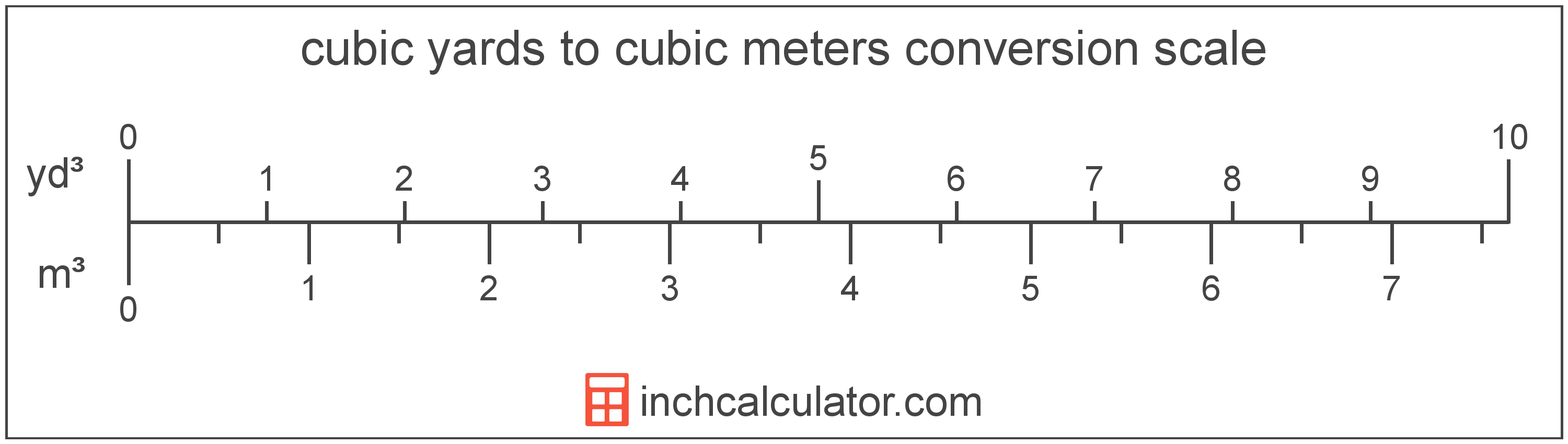# Cubic Meters to Cubic Yards Converter

Enter the volume in cubic meters below to get the value converted to cubic yards.

(find cubic meters)
Results in Cubic Yards:1 m³ = 1.307951 yd³

Do you want to convert cubic yards to cubic meters?

## How to Convert Cubic Meters to Cubic Yards

To convert a measurement in cubic meters to a measurement in cubic yards, multiply the volume by the following conversion ratio: 1.307951 cubic yards/cubic meter.

Since one cubic meter is equal to 1.307951 cubic yards, you can use this simple formula to convert:

cubic yards = cubic meters × 1.307951

The volume in cubic yards is equal to the volume in cubic meters multiplied by 1.307951.

For example, here's how to convert 5 cubic meters to cubic yards using the formula above.
cubic yards = (5 m³ × 1.307951) = 6.539753 yd³### How Many Cubic Yards Are in a Cubic Meter?

There are 1.307951 cubic yards in a cubic meter, which is why we use this value in the formula above.

1 m³ = 1.307951 yd³

## What is a Cubic Meter?

One cubic meter is equal to the volume of a cube with each edge measuring one meter.

The cubic meter, or cubic metre, is the SI derived unit for volume in the metric system. Cubic meters can be abbreviated as , and are also sometimes abbreviated as cu m, CBM, cbm, or MTQ. For example, 1 cubic meter can be written as 1 m³, 1 cu m, 1 CBM, 1 cbm, or 1 MTQ.

You can use our cubic meters calculator to calculate the volume of a space.

## What is a Cubic Yard?

The cubic yard is a unit of volume that is equal to the space consumed by a cube with each edge measuring one yard.

The cubic yard is a US customary and imperial unit of volume. A cubic yard is sometimes also referred to as a cubic yd. Cubic yards can be abbreviated as yd³, and are also sometimes abbreviated as cu yard, cu yd, or CY. For example, 1 cubic yard can be written as 1 yd³, 1 cu yard, 1 cu yd, or 1 CY.

You can calculate volume using a cubic yardage calculator if you have the dimensions of a space or object.

## Cubic Meter to Cubic Yard Conversion Table

Table showing various cubic meter measurements converted to cubic yards.
Cubic Meters Cubic Yards
1 m³ 1.308 yd³
2 m³ 2.6159 yd³
3 m³ 3.9239 yd³
4 m³ 5.2318 yd³
5 m³ 6.5398 yd³
6 m³ 7.8477 yd³
7 m³ 9.1557 yd³
8 m³ 10.46 yd³
9 m³ 11.77 yd³
10 m³ 13.08 yd³
11 m³ 14.39 yd³
12 m³ 15.7 yd³
13 m³ 17 yd³
14 m³ 18.31 yd³
15 m³ 19.62 yd³
16 m³ 20.93 yd³
17 m³ 22.24 yd³
18 m³ 23.54 yd³
19 m³ 24.85 yd³
20 m³ 26.16 yd³
21 m³ 27.47 yd³
22 m³ 28.77 yd³
23 m³ 30.08 yd³
24 m³ 31.39 yd³
25 m³ 32.7 yd³
26 m³ 34.01 yd³
27 m³ 35.31 yd³
28 m³ 36.62 yd³
29 m³ 37.93 yd³
30 m³ 39.24 yd³
31 m³ 40.55 yd³
32 m³ 41.85 yd³
33 m³ 43.16 yd³
34 m³ 44.47 yd³
35 m³ 45.78 yd³
36 m³ 47.09 yd³
37 m³ 48.39 yd³
38 m³ 49.7 yd³
39 m³ 51.01 yd³
40 m³ 52.32 yd³